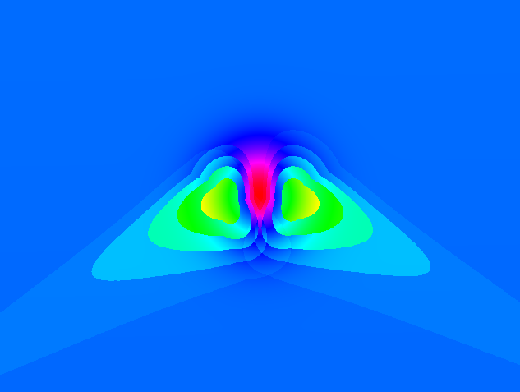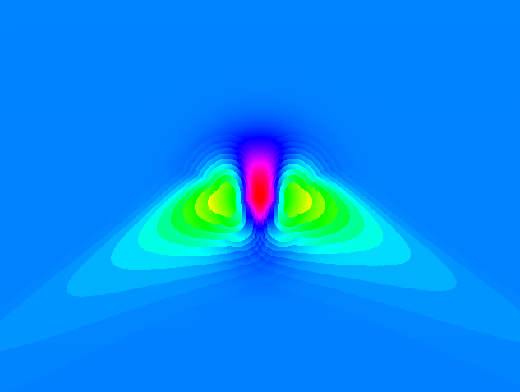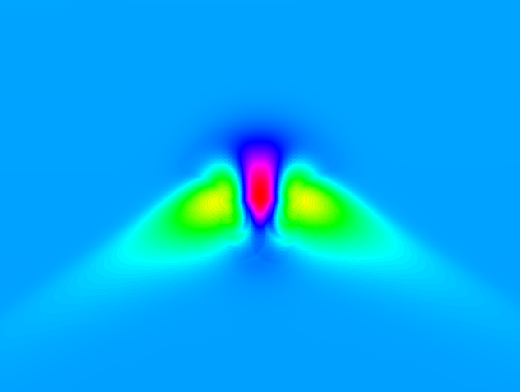R.sun

The printable version is no longer supported and may have rendering errors. Please update your browser bookmarks and please use the default browser print function instead.

Tips

The speed of r.sun is much higher if r.horizon is used first and the resulting maps are given as input to r.sun. Background: the horizon needs to be computed only one time before, not in every step within r.sun. See the example at the end of the r.sun help page.

Testing

Create an artificial surface containing a Gaussian mound:

r.surf.volcano out=gauss method=gaussian kurtosis=1

Overlay some 200m contours to show underlying topography:

r.contour in=gauss out=gauss_200m_contours step=200
d.vect gauss_200m_contours color=white

Set map's color table to highlight detail:

Time step

The following three images demonstrate the effects of using different time step parameters.Default 30 minute time step over a Gaussian mound.15 minute time step over a Gaussian mound.3 minute time step over a Gaussian mound.

The 3 minute time step takes roughly ten times as long to run as the 30 minute timestep.

Seed maps

Using seed maps can greatly speed up processing. This is especially important if you will batch process e.g. for every day of the year.

• aspin= and slopein= options: create slope and aspect maps with the r.slope.aspect module.
Caution: currently buggy. See trac #498.
Estimated speedup:  ?%.

• horizon= and horizonstep= options: Pre-calculate horizon shadows by creating a series of horizon rasters with the r.horizon module.
Results not as smooth as without using this option?? See trac #498.
Estimated speedup:  ?%.

• latin= and longin= options: Pre-calculate latitudes and longitudes for each raster cell.

The following script will create a raster containing latitude as the raster value:

# create latitude map (WGS84)
g.region rast=elevation.dem
r.mapcalc one=1
r.out.xyz one | \
cut -f1,2 -d'|' | \
m.proj -oed --quiet | \
sed -e 's/[ \t]/|/g' | \
cut -f1-4 -d'|' | \
r.in.xyz in=- z=4 out=elevation.lat
g.remove one

Creating the longitude map is exactly the same but use column 3 of the m.proj output instead of column 4:

# create longitude map (WGS84)
g.region rast=elevation.dem
r.mapcalc one=1
r.out.xyz one | \
cut -f1,2 -d'|' | \
m.proj -oed --quiet | \
sed -e 's/[ \t]/|/g' | \
cut -f1-4 -d'|' | \
r.in.xyz in=- z=3 out=elevation.lon
g.remove one
Estimated speedup: 1.3% (in one test).
See trac #498.

The above assumes that your projection's ellipsoid is WGS84. If it isn't, use other proj_out= terms with m.proj as required instead of the -o flag.

Automation

It can be tedious to set up and run a long series of r.sun simulations for every day of the year. To automate this we can write a small shell script loop:

for DAY in `seq 1 365` ; do
DAY_STR=`echo \$DAY | awk '{printf("%.03d", \$1)}'`
echo "Processing day \$DAY_STR at `date` ..."

r.sun -s elevin=elevation.dem day=\$DAY lin=\$LINKE step=0.05 \
done
• g.linke_by_day.py is a small program which will return the Linke coefficient for any day of the year, interpolated from monthly values. You need to edit the script to fill in values appropriate for your study area.
• If you have a multi-core CPU and you'd like to speed things up, here is a small Bourne shell script implementing a poor-man's multi-processing trick:
### r.sun mode 2 loop ###
BEGIN=1
END=365
STEP=1
NUM_CORES=4

for DAY in `seq \$BEGIN \$STEP \$END` ; do
DAY_STR=`echo \$DAY | awk '{printf("%.03d", \$1)}'`
echo "Processing day \$DAY_STR at `date` ..."

CMD="r.sun -s elevin=elevation.dem day=\$DAY lin=\$LINKE step=0.05 \

# poor man's multi-threading for a multi-core CPU
MODULUS=`echo "\$DAY \$STEP \$NUM_CORES" | awk '{print \$1 % (\$2 * \$3)}'`
if [ "\$MODULUS" = "\$STEP" ] ; then
# stall to let the background jobs finish
\$CMD
sleep 2
#while [ `pgrep -c r.sun` -ne 0 ] ; do
#   sleep 5
#done
else
\$CMD &
fi
done
• For a r.sun Mode 1 loop, see the OpenMP page.

ToDo

• Add support for OpenMP parallelization